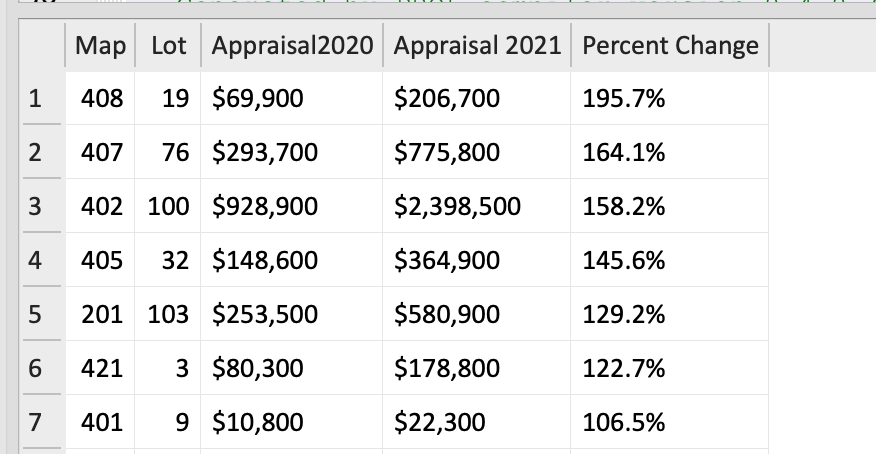# Format pretty reports

richb-hanover· ·
Updated

I can no longer bring myself to write bare SQL - PRQL makes building queries so easy. So here’s how I use PRQL functions and aliases for naming variables to “pretty up” a SQL report.

I have an SQLite database with a table named `PropertyData` and I want to show the change of column `App_Total2020` and `App_Total2021` across years, displaying their values along with their percent change.

## Functions

Of course, property values are in dollars. So I could simply display them as `450000`. But they are more compelling if they’re written as `\$450,000`. Writing the SQL code and format strings for each column would be tedious and error-prone. PRQL allows me to create a `dollars` function and then use it multiple times:

``````# dollars displays a numeric value as whole dollars with commas
let dollars = d -> s"""printf("\$%,d",{d})"""

select {
(dollars App_Total2020),
(dollars App_Total2021),
}
``````

I also want to compute the percent change between values. It’s easy to create a `percent_diff` function:

``````# percent_diff computes the amount (percent) the new differs from old
let percent_diff = old new -> 100.0*( new - old ) / old
``````

One final function: the `percent_diff` function returns a floating point number with many digits after the decimal place. I only want to display one place in my results, with a trailing `%`. So I wrote a `format_percent` function that uses a `printf()` to format the value.

``````# format_percent prints a floating point number with "%"
let format_percent = v -> s'printf("%1.1f%", {v})'
``````

Use a PRQL alias to assign each column a nice name. This becomes its column heading. The examples above might be:

``````select [
Appraisal2020 = (dollars App_Total2020),
`Appraisal 2021` = (dollars App_Total2021),
]
``````

Note how the second example puts the column heading in backticks to preserve spaces.

## Excluding certain columns

I want to sort results by the (numeric) percent change, but I don’t want to display that percentage value (with multiple decimal places) in the final table. So I split the query into pieces: the first `select` collects all the necessary columns, adding a new column using `percent_diff`. The query then sorts the values and passes those results to a second `select` that’s responsible for formatting the column headings and contents (using aliases and `format_percent`).

## Putting it all together

Here is my workflow for a typical query using the tools listed below: 1

• Enter the PRQL query in the VS Code editor. Use a `.prql` suffix for the file.
• Open the PRQL VS Code extension (Ctl-Shift-P, or Cmd-Shift-P on Mac). It’ll appear and display the compiled SQL in a second pane on the right.
• Copy the SQL from the right pane and paste it into the DBM program. Run the query. That’s it!

Here’s the PRQL for this example, followed by the result from my database. Note: Paste the PRQL query below into the Playground to see what the PRQL compiler produces.

``````# dollars displays a numeric value as dollars with commas
let dollars = d -> s"""printf("\$%,d",{d})"""

# percent_diff computes the amount (percent) the new differs from old
let percent_diff = old new -> 100.0*( new - old ) / old

# format_percent prints a floating point number with "%"
let format_percent = v -> s'printf("%1.1f%", {v})'

# Step 1: First calculate important columns
from PropertyData
select {
Map, Lot,
App_Total2020,
App_Total2021,
pct_change = (percent_diff App_Total2020 App_Total2021),
}

# Step 2: Sort the resulting table by pct_change
sort [-pct_change]

# Step 3: Format the column headings and contents
select [
Map, Lot,
Appraisal2020 = (dollars App_Total2020),
`Appraisal 2021` = (dollars App_Total2021),
`Percent Change` = (format_percent pct_change),
]
take 20
``````

The image below shows the result of that query:

• the column headings match the aliases of the second `select` statement,
• the `dollars` function formats values with `\$` and `,` as expected,
• the `percent_diff` function computes the percent change between the old and new values,
• the `format_percent` function formats the value with a single decimal place and appends a `%`.1. My Tools: I use Visual Studio Code to maintain a folder of PRQL queries for re-use. I use the PRQL VS Code extension to compile PRQL into SQL. My data is in a SQLite database, and I use DB Browser for SQLite to run queries. ↩︎